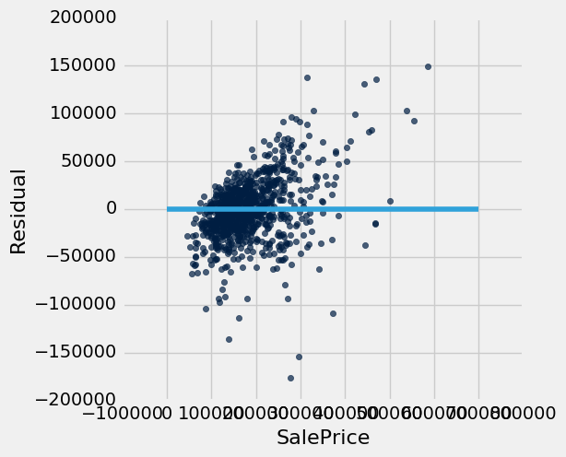# 十五、分类

David Wagner 是这一章的主要作者。

• 他们有一些每个订单的信息（例如，它的总值，订单是否被运送到这个客户以前使用过的地址，是否与信用卡持有人的账单地址相同）。他们有很多过去的订单数据，他们知道哪些过去的订单是欺诈性的，哪些不是。他们想要学习规律，这将帮助他们预测新订单到达时，这些新订单是否有欺诈行为。

• 在线约会网站希望预测：这两个人合适吗？他们有很多数据，他们过去向顾客推荐一些东西，它们就知道了哪个是成功的。当新客户注册时，他们想预测谁可能是他们的最佳伴侣。

• 医生想知道：这个病人是否患有癌症？根据一些实验室测试的结果，他们希望能够预测特定患者是否患有癌症。基于一些实验室测试的测量结果，以及他们是否最终发展成癌症，并且由此他们希望尝试推断，哪些测量结果倾向于癌症（或非癌症）特征，以便能够准确地诊断未来的患者。

• 政客们想预测：你打算为他们投票吗？这将帮助他们将筹款工作集中在可能支持他们的人身上，并将动员工作集中在投票给他们的人身上。公共数据库和商业数据库有大多数人的大量信息，例如，他们是否拥有房屋或房租；他们是否住在富裕的社区还是贫穷的社区；他们的兴趣和爱好；他们的购物习惯；等等。政治团体已经调查了一些选民，并找到了他们计划投票的人，所以他们有一些正确答案已知的例子。

## 最近邻

### 慢性肾病

``````ckd = Table.read_table('ckd.csv').relabeled('Blood Glucose Random', 'Glucose')
ckd
``````
Age Blood Pressure Specific Gravity Albumin Sugar Red Blood Cells Pus Cell Pus Cell clumps Bacteria Glucose Blood Urea Serum Creatinine Sodium Potassium Hemoglobin Packed Cell Volume White Blood Cell Count Red Blood Cell Count Hypertension Diabetes Mellitus Coronary Artery Disease Appetite Pedal Edema Anemia Class
48 70 1.005 4 0 normal abnormal present notpresent 117 56 3.8 111 2.5 11.2 32 6700 3.9 yes no no poor yes yes 1
53 90 1.02 2 0 abnormal abnormal present notpresent 70 107 7.2 114 3.7 9.5 29 12100 3.7 yes yes no poor no yes 1
63 70 1.01 3 0 abnormal abnormal present notpresent 380 60 2.7 131 4.2 10.8 32 4500 3.8 yes yes no poor yes no 1
68 80 1.01 3 2 normal abnormal present present 157 90 4.1 130 6.4 5.6 16 11000 2.6 yes yes yes poor yes no 1
61 80 1.015 2 0 abnormal abnormal notpresent notpresent 173 148 3.9 135 5.2 7.7 24 9200 3.2 yes yes yes poor yes yes 1
48 80 1.025 4 0 normal abnormal notpresent notpresent 95 163 7.7 136 3.8 9.8 32 6900 3.4 yes no no good no yes 1
69 70 1.01 3 4 normal abnormal notpresent notpresent 264 87 2.7 130 4 12.5 37 9600 4.1 yes yes yes good yes no 1
73 70 1.005 0 0 normal normal notpresent notpresent 70 32 0.9 125 4 10 29 18900 3.5 yes yes no good yes no 1
73 80 1.02 2 0 abnormal abnormal notpresent notpresent 253 142 4.6 138 5.8 10.5 33 7200 4.3 yes yes yes good no no 1
46 60 1.01 1 0 normal normal notpresent notpresent 163 92 3.3 141 4 9.8 28 14600 3.2 yes yes no good no no 1

（省略了 148 行）

``````ckd = Table().with_columns(
'Hemoglobin', standard_units(ckd.column('Hemoglobin')),
'Glucose', standard_units(ckd.column('Glucose')),
'White Blood Cell Count', standard_units(ckd.column('White Blood Cell Count')),
'Class', ckd.column('Class')
)
ckd
``````
Hemoglobin Glucose White Blood Cell Count Class
-0.865744 -0.221549 -0.569768 1
-1.45745 -0.947597 1.16268 1
-1.00497 3.84123 -1.27558 1
-2.81488 0.396364 0.809777 1
-2.08395 0.643529 0.232293 1
-1.35303 -0.561402 -0.505603 1
-0.413266 2.04928 0.360623 1
-1.28342 -0.947597 3.34429 1
-1.10939 1.87936 -0.409356 1
-1.35303 0.489051 1.96475 1

（省略了 148 行）

``````color_table = Table().with_columns(
'Class', make_array(1, 0),
'Color', make_array('darkblue', 'gold')
)
ckd = ckd.join('Class', color_table)
ckd.scatter('Hemoglobin', 'Glucose', colors='Color')
``````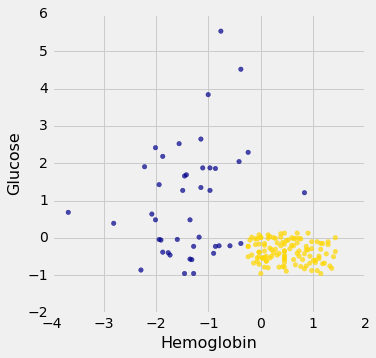### 最近邻分类器

``````# In this example, Alice's Hemoglobin attribute is 0 and her Glucose is 1.5.
alice = make_array(0, 1.5)
show_closest(alice)
``````• 找到训练集中离新点最近的点。
• 如果最近的点是“CKD”点，则将新点划分为“CKD”。如果最近的点是“非 CKD”点，则将新点划分为“非 CKD”。

## 决策边界

``````alice = make_array(0, 0.97)
show_closest(alice)
``````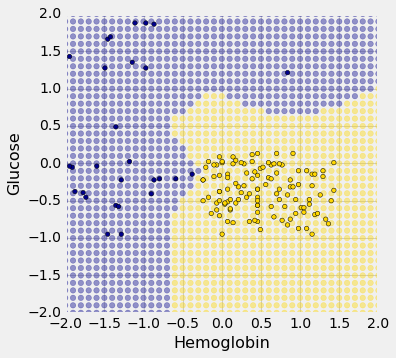## KNN

``````ckd.scatter('White Blood Cell Count', 'Glucose', colors='Color')
``````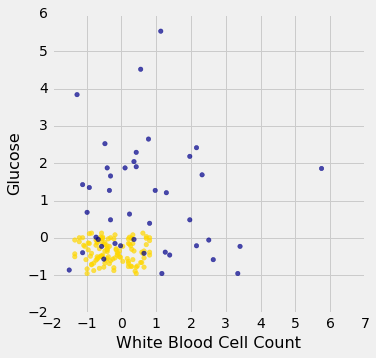## 过于乐观的“测试”

``````ckd.scatter('White Blood Cell Count', 'Glucose', colors='Color')
``````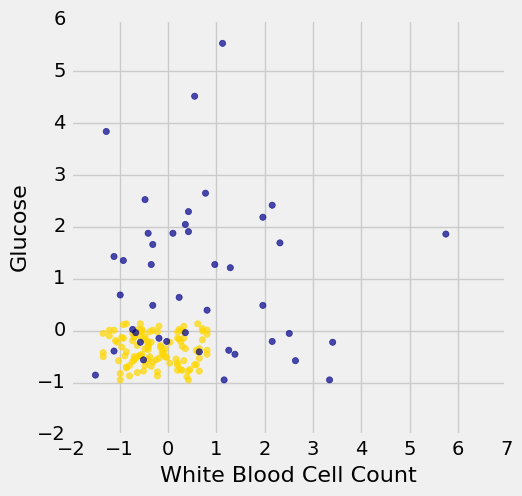### 生成测试集

• 训练集，我们可以对它进行任何大量的探索来建立我们的分类器
• 一个单独的测试集，在它上面测试我们的分类器，看看分类的正确比例是多少
• 个体的底层总体，我们不了解它；我们的希望是我们的分类器对于这些个体也会成功，就像我们的测试集一样。

`ckd`有 158 个个体。让我们将它们随机的一半用于训练，另一半用于测试。为此，我们将打乱所有行，把前 79 个作为训练集，其余的 79 个用于测试。

``````shuffled_ckd = ckd.sample(with_replacement=False)
training = shuffled_ckd.take(np.arange(79))
testing = shuffled_ckd.take(np.arange(79, 158))
``````

``````training.scatter('White Blood Cell Count', 'Glucose', colors='Color')
plt.xlim(-2, 6)
plt.ylim(-2, 6);
``````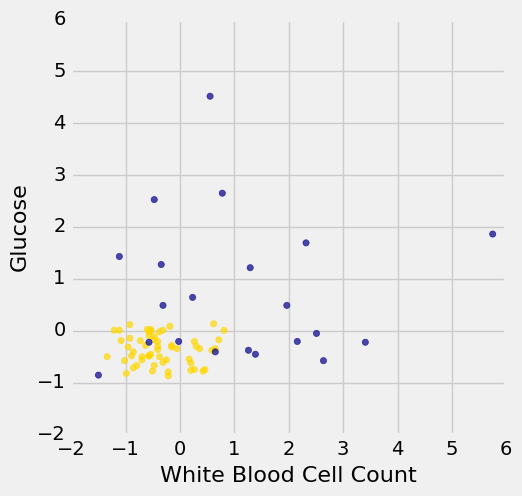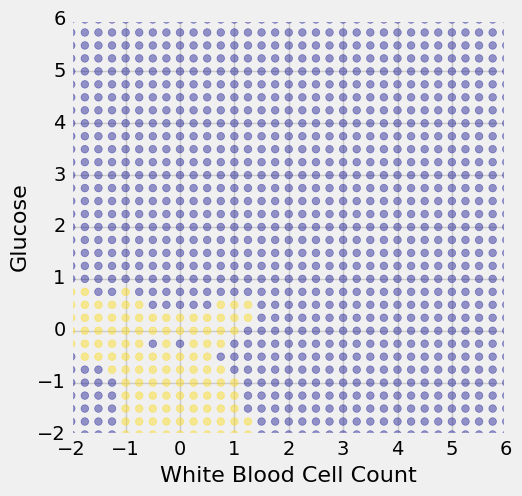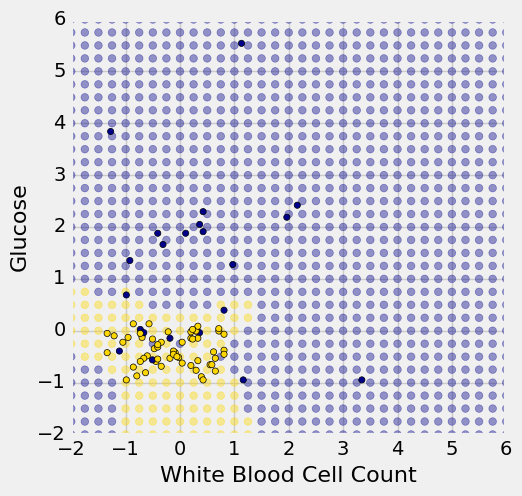## 表的行

``````ckd = Table.read_table('ckd.csv').relabeled('Blood Glucose Random', 'Glucose')
``````

``````ckd.row(0)
Row(Age=48, Blood Pressure=70, Specific Gravity=1.0049999999999999, Albumin=4, Sugar=0, Red Blood Cells='normal', Pus Cell='abnormal', Pus Cell clumps='present', Bacteria='notpresent', Glucose=117, Blood Urea=56, Serum Creatinine=3.7999999999999998, Sodium=111, Potassium=2.5, Hemoglobin=11.199999999999999, Packed Cell Volume=32, White Blood Cell Count=6700, Red Blood Cell Count=3.8999999999999999, Hypertension='yes', Diabetes Mellitus='no', Coronary Artery Disease='no', Appetite='poor', Pedal Edema='yes', Anemia='yes', Class=1)
``````

``````ckd.row(0).item(3)
4
``````

### 将行转换为数组（可能的时候）

``````ckd = Table().with_columns(
'Hemoglobin', standard_units(ckd.column('Hemoglobin')),
'Glucose', standard_units(ckd.column('Glucose')),
'Class', ckd.column('Class')
)

color_table = Table().with_columns(
'Class', make_array(1, 0),
'Color', make_array('darkblue', 'gold')
)
ckd = ckd.join('Class', color_table)
ckd
``````
Class Hemoglobin Glucose Color
0 0.456884 0.133751 gold
0 1.153 -0.947597 gold
0 0.770138 -0.762223 gold
0 0.596108 -0.190654 gold
0 -0.239236 -0.49961 gold
0 -0.0304002 -0.159758 gold
0 0.282854 -0.00527964 gold
0 0.108824 -0.623193 gold
0 0.0740178 -0.515058 gold
0 0.83975 -0.422371 gold

（省略了 148 行）

``````alice = make_array(0, 1.1)
ckd.scatter('Hemoglobin', 'Glucose', colors='Color')
plots.scatter(alice.item(0), alice.item(1), color='red', s=30);
``````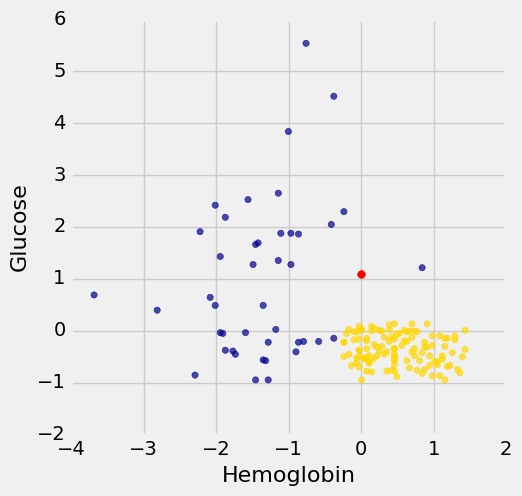``````ckd_attributes = ckd.select('Hemoglobin', 'Glucose')
ckd_attributes
``````
Hemoglobin Glucose
0.456884 0.133751
1.153 -0.947597
0.770138 -0.762223
0.596108 -0.190654
-0.239236 -0.49961
-0.0304002 -0.159758
0.282854 -0.00527964
0.108824 -0.623193
0.0740178 -0.515058
0.83975 -0.422371

（省略了 148 行）

``````ckd_attributes.row(3)
Row(Hemoglobin=0.59610766482326683, Glucose=-0.19065363034327712)
np.array(ckd_attributes.row(3))
array([ 0.59610766, -0.19065363])
``````

### 只有两个属性时点的距离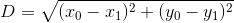（这是从哪里来的？它来自勾股定理：我们有一个直角三角形，边长为`x0 - x1``y0 - y1`，我们想要求出斜边的长度。）

``````patient3 = np.array(ckd_attributes.row(3))
alice, patient3
(array([ 0. ,  1.1]), array([ 0.59610766, -0.19065363]))
distance = np.sqrt(np.sum((alice - patient3)**2))
distance
1.4216649188818471
``````

``````def distance(point1, point2):
"""Returns the Euclidean distance between point1 and point2.

Each argument is an array containing the coordinates of a point."""
return np.sqrt(np.sum((point1 - point2)**2))
distance(alice, patient3)
1.4216649188818471
``````

### 在整个行上使用`apply`

``````t = ckd_attributes.take(np.arange(5))
t
``````
Hemoglobin Glucose
0.456884 0.133751
1.153 -0.947597
0.770138 -0.762223
0.596108 -0.190654
-0.239236 -0.49961

``````def max_abs(row):
return np.max(np.abs(np.array(row)))
max_abs(t.row(4))
0.49961028259186968
``````

``````t.apply(max_abs)
array([ 0.4568837 ,  1.15300352,  0.77013762,  0.59610766,  0.49961028])
``````

### Alice 的 K 最近邻

• 步骤 1：的是 Alice 与训练样本中每个点之间的距离。
• 步骤 2：按照距离的升序对数据表进行排序。
• 步骤 3：取得有序表的前 5 行。

``````alice
array([ 0. ,  1.1])
``````

``````def distance_from_alice(row):
"""Returns distance between Alice and a row of the attributes table"""
return distance(alice, np.array(row))
distance_from_alice(ckd_attributes.row(3))
1.4216649188818471
``````

``````distances = ckd_attributes.apply(distance_from_alice)
ckd_with_distances = ckd.with_column('Distance from Alice', distances)
ckd_with_distances
``````
Class Hemoglobin Glucose Color Distance from Alice
0 0.456884 0.133751 gold 1.06882
0 1.153 -0.947597 gold 2.34991
0 0.770138 -0.762223 gold 2.01519
0 0.596108 -0.190654 gold 1.42166
0 -0.239236 -0.49961 gold 1.6174
0 -0.0304002 -0.159758 gold 1.26012
0 0.282854 -0.00527964 gold 1.1409
0 0.108824 -0.623193 gold 1.72663
0 0.0740178 -0.515058 gold 1.61675
0 0.83975 -0.422371 gold 1.73862

（省略了 148 行）

``````sorted_by_distance = ckd_with_distances.sort('Distance from Alice')
sorted_by_distance
``````
Class Hemoglobin Glucose Color Distance from Alice
1 0.83975 1.2151 darkblue 0.847601
1 -0.970162 1.27689 darkblue 0.986156
0 -0.0304002 0.0874074 gold 1.01305
0 0.14363 0.0874074 gold 1.02273
1 -0.413266 2.04928 darkblue 1.03534
0 0.387272 0.118303 gold 1.05532
0 0.456884 0.133751 gold 1.06882
0 0.178436 0.0410639 gold 1.07386
0 0.00440582 0.025616 gold 1.07439
0 -0.169624 0.025616 gold 1.08769

（省略了 148 行）

``````alice_5_nearest_neighbors = sorted_by_distance.take(np.arange(5))
alice_5_nearest_neighbors
``````
Class Hemoglobin Glucose Color Distance from Alice
1 0.83975 1.2151 darkblue 0.847601
1 -0.970162 1.27689 darkblue 0.986156
0 -0.0304002 0.0874074 gold 1.01305
0 0.14363 0.0874074 gold 1.02273
1 -0.413266 2.04928 darkblue 1.03534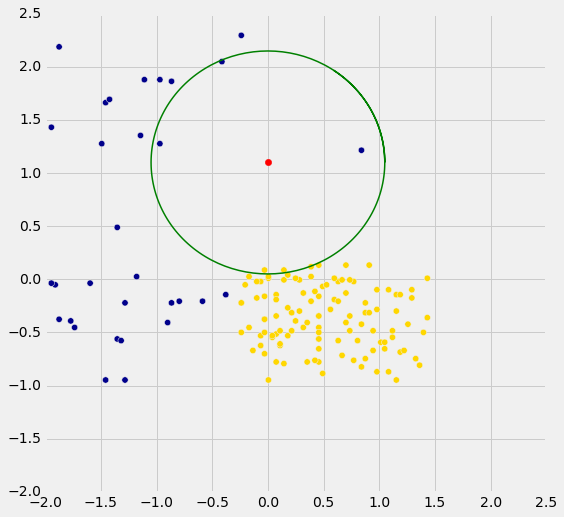## 实现分类器

### 钞票检测

``````banknotes = Table.read_table('banknote.csv')
banknotes
``````
WaveletVar WaveletSkew WaveletCurt Entropy Class
3.6216 8.6661 -2.8073 -0.44699 0
4.5459 8.1674 -2.4586 -1.4621 0
3.866 -2.6383 1.9242 0.10645 0
3.4566 9.5228 -4.0112 -3.5944 0
0.32924 -4.4552 4.5718 -0.9888 0
4.3684 9.6718 -3.9606 -3.1625 0
3.5912 3.0129 0.72888 0.56421 0
2.0922 -6.81 8.4636 -0.60216 0
3.2032 5.7588 -0.75345 -0.61251 0
1.5356 9.1772 -2.2718 -0.73535 0

（省略了 1362 行）

``````color_table = Table().with_columns(
'Class', make_array(1, 0),
'Color', make_array('darkblue', 'gold')
)
banknotes = banknotes.join('Class', color_table)
banknotes.scatter('WaveletVar', 'WaveletCurt', colors='Color')
``````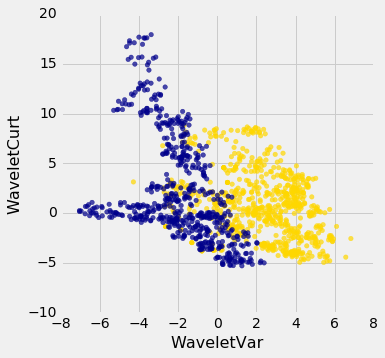``````banknotes.scatter('WaveletSkew', 'Entropy', colors='Color')
``````### 多个属性

``````ax = plt.figure(figsize=(8,8)).add_subplot(111, projection='3d')
ax.scatter(banknotes.column('WaveletSkew'),
banknotes.column('WaveletVar'),
banknotes.column('WaveletCurt'),
c=banknotes.column('Color'));
``````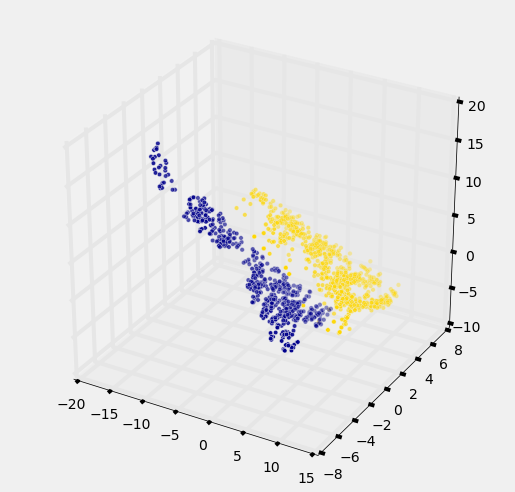### 多维距离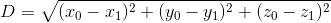``````def distance(point1, point2):
"""Returns the distance between point1 and point2
where each argument is an array
consisting of the coordinates of the point"""
return np.sqrt(np.sum((point1 - point2)**2))
``````

``````wine = Table.read_table('wine.csv')

# For converting Class to binary

def is_one(x):
if x == 1:
return 1
else:
return 0

wine = wine.with_column('Class', wine.apply(is_one, 0))
wine
``````
Class Alcohol Malic Acid Ash Alcalinity of Ash Magnesium Total Phenols Flavanoids Nonflavanoid phenols Proanthocyanins Color Intensity Hue OD280/OD315 of diulted wines Proline
1 14.23 1.71 2.43 15.6 127 2.8 3.06 0.28 2.29 5.64 1.04 3.92 1065
1 13.2 1.78 2.14 11.2 100 2.65 2.76 0.26 1.28 4.38 1.05 3.4 1050
1 13.16 2.36 2.67 18.6 101 2.8 3.24 0.3 2.81 5.68 1.03 3.17 1185
1 14.37 1.95 2.5 16.8 113 3.85 3.49 0.24 2.18 7.8 0.86 3.45 1480
1 13.24 2.59 2.87 21 118 2.8 2.69 0.39 1.82 4.32 1.04 2.93 735
1 14.2 1.76 2.45 15.2 112 3.27 3.39 0.34 1.97 6.75 1.05 2.85 1450
1 14.39 1.87 2.45 14.6 96 2.5 2.52 0.3 1.98 5.25 1.02 3.58 1290
1 14.06 2.15 2.61 17.6 121 2.6 2.51 0.31 1.25 5.05 1.06 3.58 1295
1 14.83 1.64 2.17 14 97 2.8 2.98 0.29 1.98 5.2 1.08 2.85 1045
1 13.86 1.35 2.27 16 98 2.98 3.15 0.22 1.85 7.22 1.01 3.55 1045

``````wine_attributes = wine.drop('Class')
distance(np.array(wine_attributes.row(0)), np.array(wine_attributes.row(1)))
31.265012394048398
``````

``````distance(np.array(wine_attributes.row(0)), np.array(wine_attributes.row(177)))
506.05936766351834
``````

``````wine_with_colors = wine.join('Class', color_table)
wine_with_colors.scatter('Flavanoids', 'Alcohol', colors='Color')
``````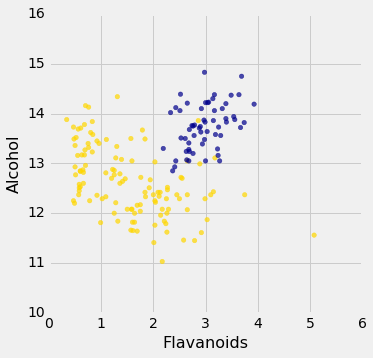``````wine_with_colors.scatter('Alcalinity of Ash', 'Ash', colors='Color')
``````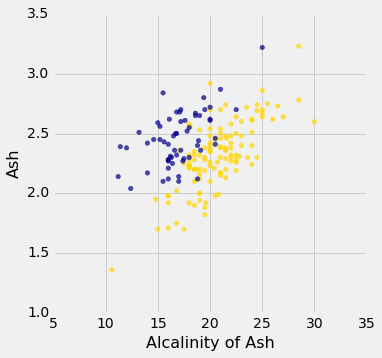``````wine_with_colors.scatter('Magnesium', 'Total Phenols', colors='Color')
``````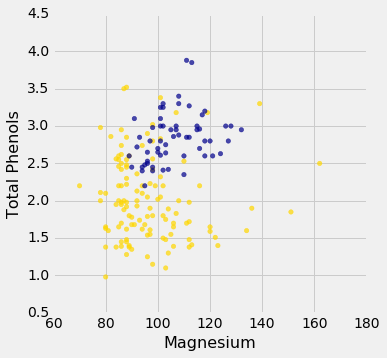### 实现计划

``````def closest(training, p, k):
...

def majority(topkclasses):
...

def classify(training, p, k):
kclosest = closest(training, p, k)
kclosest.classes = kclosest.select('Class')
return majority(kclosest)
``````

### 实现步骤 1

``````def distance(point1, point2):
"""Returns the distance between point1 and point2
where each argument is an array
consisting of the coordinates of the point"""
return np.sqrt(np.sum((point1 - point2)**2))

def all_distances(training, new_point):
"""Returns an array of distances
between each point in the training set
and the new point (which is a row of attributes)"""
attributes = training.drop('Class')
def distance_from_point(row):
return distance(np.array(new_point), np.array(row))
return attributes.apply(distance_from_point)

def table_with_distances(training, new_point):
"""Augments the training table
with a column of distances from new_point"""
return training.with_column('Distance', all_distances(training, new_point))

def closest(training, new_point, k):
"""Returns a table of the k rows of the augmented table
corresponding to the k smallest distances"""
with_dists = table_with_distances(training, new_point)
sorted_by_distance = with_dists.sort('Distance')
topk = sorted_by_distance.take(np.arange(k))
``````

``````special_wine = wine.drop('Class').row(0)
``````

``````closest(wine, special_wine, 5)
``````
Class Alcohol Malic Acid Ash Alcalinity of Ash Magnesium Total Phenols Flavanoids Nonflavanoid phenols Proanthocyanins Color Intensity Hue OD280/OD315 of diulted wines Proline Distance
1 14.23 1.71 2.43 15.6 127 2.8 3.06 0.28 2.29 5.64 1.04 3.92 1065 0
1 13.74 1.67 2.25 16.4 118 2.6 2.9 0.21 1.62 5.85 0.92 3.2 1060 10.3928
1 14.21 4.04 2.44 18.9 111 2.85 2.65 0.3 1.25 5.24 0.87 3.33 1080 22.3407
1 14.1 2.02 2.4 18.8 103 2.75 2.92 0.32 2.38 6.2 1.07 2.75 1060 24.7602
1 14.38 3.59 2.28 16 102 3.25 3.17 0.27 2.19 4.9 1.04 3.44 1065 25.0947

### 实现步骤 2 和 3

``````def majority(topkclasses):
ones = topkclasses.where('Class', are.equal_to(1)).num_rows
zeros = topkclasses.where('Class', are.equal_to(0)).num_rows
if ones > zeros:
return 1
else:
return 0

def classify(training, new_point, k):
closestk = closest(training, new_point, k)
topkclasses = closestk.select('Class')
return majority(topkclasses)
classify(wine, special_wine, 5)
1
``````

``````special_wine = wine.drop('Class').row(177)
classify(wine, special_wine, 5)
0
``````

## 分类器的准确性

### 测量我们的葡萄酒分类器的准确率

``````shuffled_wine = wine.sample(with_replacement=False)
training_set = shuffled_wine.take(np.arange(89))
test_set  = shuffled_wine.take(np.arange(89, 178))
``````

``````def count_zero(array):
"""Counts the number of 0's in an array"""
return len(array) - np.count_nonzero(array)

def count_equal(array1, array2):
"""Takes two numerical arrays of equal length
and counts the indices where the two are equal"""
return count_zero(array1 - array2)

def evaluate_accuracy(training, test, k):
test_attributes = test.drop('Class')
def classify_testrow(row):
return classify(training, row, k)
c = test_attributes.apply(classify_testrow)
return count_equal(c, test.column('Class')) / test.num_rows
``````

``````evaluate_accuracy(training_set, test_set, 5)
0.9213483146067416
``````

### 乳腺癌诊断

``````patients = Table.read_table('breast-cancer.csv').drop('ID')
patients
``````
Clump Thickness Uniformity of Cell Size Uniformity of Cell Shape Marginal Adhesion Single Epithelial Cell Size Bare Nuclei Bland Chromatin Normal Nucleoli Mitoses Class
5 1 1 1 2 1 3 1 1 0
5 4 4 5 7 10 3 2 1 0
3 1 1 1 2 2 3 1 1 0
6 8 8 1 3 4 3 7 1 0
4 1 1 3 2 1 3 1 1 0
8 10 10 8 7 10 9 7 1 1
1 1 1 1 2 10 3 1 1 0
2 1 2 1 2 1 3 1 1 0
2 1 1 1 2 1 1 1 5 0
4 2 1 1 2 1 2 1 1 0

（省略了 673 行）

``````color_table = Table().with_columns(
'Class', make_array(1, 0),
'Color', make_array('darkblue', 'gold')
)
patients_with_colors = patients.join('Class', color_table)
patients_with_colors.scatter('Bland Chromatin', 'Single Epithelial Cell Size', colors='Color')
``````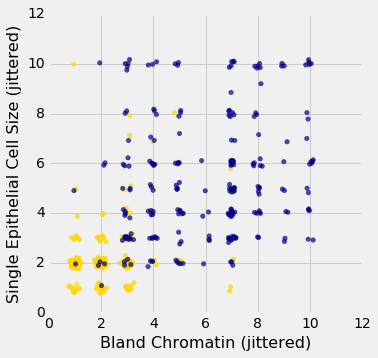``````shuffled_patients = patients.sample(683, with_replacement=False)
training_set = shuffled_patients.take(np.arange(342))
test_set  = shuffled_patients.take(np.arange(342, 683))
``````

``````evaluate_accuracy(training_set, test_set, 5)
0.967741935483871
``````

## 多元回归

### 房价

``````all_sales = Table.read_table('house.csv')
sales = all_sales.where('Bldg Type', '1Fam').where('Sale Condition', 'Normal').select(
'SalePrice', '1st Flr SF', '2nd Flr SF',
'Total Bsmt SF', 'Garage Area',
'Wood Deck SF', 'Open Porch SF', 'Lot Area',
'Year Built', 'Yr Sold')
sales.sort('SalePrice')
``````
SalePrice 1st Flr SF 2nd Flr SF Total Bsmt SF Garage Area Wood Deck SF Open Porch SF Lot Area Year Built Yr Sold
35000 498 0 498 216 0 0 8088 1922 2006
39300 334 0 0 0 0 0 5000 1946 2007
40000 649 668 649 250 0 54 8500 1920 2008
45000 612 0 0 308 0 0 5925 1940 2009
52000 729 0 270 0 0 0 4130 1935 2008
52500 693 0 693 0 0 20 4118 1941 2006
55000 723 363 723 400 0 24 11340 1920 2008
55000 796 0 796 0 0 0 3636 1922 2008
57625 810 0 0 280 119 24 21780 1910 2009
58500 864 0 864 200 0 0 8212 1914 2010

（省略了 1992 行）

``````sales.hist('SalePrice', bins=32, unit='\$')
``````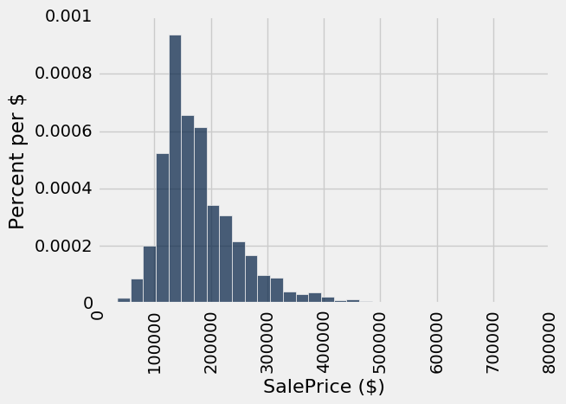### 相关性

``````sales.scatter('1st Flr SF', 'SalePrice')

correlation(sales, 'SalePrice', '1st Flr SF')
0.64246625410302249
``````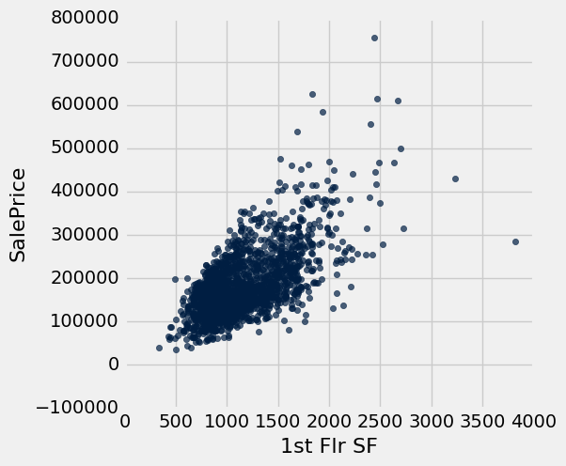``````for label in sales.labels:
print('Correlation of', label, 'and SalePrice:\t', correlation(sales, label, 'SalePrice'))
Correlation of SalePrice and SalePrice:     1.0
Correlation of 1st Flr SF and SalePrice:     0.642466254103
Correlation of 2nd Flr SF and SalePrice:     0.35752189428
Correlation of Total Bsmt SF and SalePrice:     0.652978626757
Correlation of Garage Area and SalePrice:     0.638594485252
Correlation of Wood Deck SF and SalePrice:     0.352698666195
Correlation of Open Porch SF and SalePrice:     0.336909417026
Correlation of Lot Area and SalePrice:     0.290823455116
Correlation of Year Built and SalePrice:     0.565164753714
Correlation of Yr Sold and SalePrice:     0.0259485790807
``````

``````both_floors = sales.column(1) + sales.column(2)
correlation(sales.with_column('Both Floors', both_floors), 'SalePrice', 'Both Floors')
0.7821920556134877
``````

## 多元线性回归

``````train, test = sales.split(1001)
print(train.num_rows, 'training and', test.num_rows, 'test instances.')
1001 training and 1001 test instances.
``````

``````def predict(slopes, row):
return sum(slopes * np.array(row))

example_row = test.drop('SalePrice').row(0)
print('Predicting sale price for:', example_row)
example_slopes = np.random.normal(10, 1, len(example_row))
print('Using slopes:', example_slopes)
print('Result:', predict(example_slopes, example_row))
Predicting sale price for: Row(1st Flr SF=1092, 2nd Flr SF=1020, Total Bsmt SF=952.0, Garage Area=576.0, Wood Deck SF=280, Open Porch SF=0, Lot Area=11075, Year Built=1969, Yr Sold=2008)
Using slopes: [  9.99777721   9.019661    11.13178317   9.40645585  11.07998556
11.03830075  10.26908341  10.42534332  11.00103437]
Result: 195583.275784
``````

``````print('Actual sale price:', test.column('SalePrice').item(0))
print('Predicted sale price using random slopes:', predict(example_slopes, example_row))
Actual sale price: 206900
Predicted sale price using random slopes: 195583.275784
``````

### 最小二乘回归

``````train_prices = train.column(0)
train_attributes = train.drop(0)

def rmse(slopes, attributes, prices):
errors = []
for i in np.arange(len(prices)):
predicted = predict(slopes, attributes.row(i))
actual = prices.item(i)
errors.append((predicted - actual) ** 2)
return np.mean(errors) ** 0.5

def rmse_train(slopes):
return rmse(slopes, train_attributes, train_prices)

print('RMSE of all training examples using random slopes:', rmse_train(example_slopes))
RMSE of all training examples using random slopes: 69653.9880638
``````

``````best_slopes = minimize(rmse_train, start=example_slopes, smooth=True, array=True)
print('The best slopes for the training set:')
Table(train_attributes.labels).with_row(list(best_slopes)).show()
print('RMSE of all training examples using the best slopes:', rmse_train(best_slopes))
The best slopes for the training set:
``````
1st Flr SF 2nd Flr SF Total Bsmt SF Garage Area Wood Deck SF Open Porch SF Lot Area Year Built Yr Sold
73.7779 72.3057 51.8885 46.5581 39.3267 11.996 0.451265 538.243 -534.634
``````RMSE of all training examples using the best slopes: 31146.4442711
``````

### 解释多元线性回归

``````test_prices = test.column(0)
test_attributes = test.drop(0)

def rmse_test(slopes):
return rmse(slopes, test_attributes, test_prices)

rmse_linear = rmse_test(best_slopes)
print('Test set RMSE for multiple linear regression:', rmse_linear)
Test set RMSE for multiple linear regression: 31105.4799398
``````

``````def fit(row):
return sum(best_slopes * np.array(row))

test.with_column('Fitted', test.drop(0).apply(fit)).scatter('Fitted', 0)
plots.plot([0, 5e5], [0, 5e5]);
``````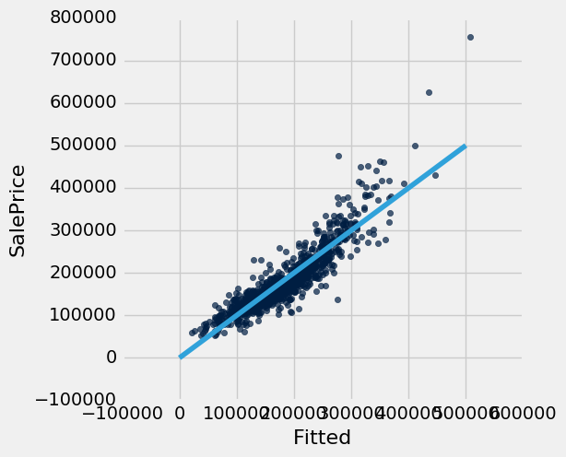``````test.with_column('Residual', test_prices-test.drop(0).apply(fit)).scatter(0, 'Residual')
plots.plot([0, 7e5], [0, 0]);
``````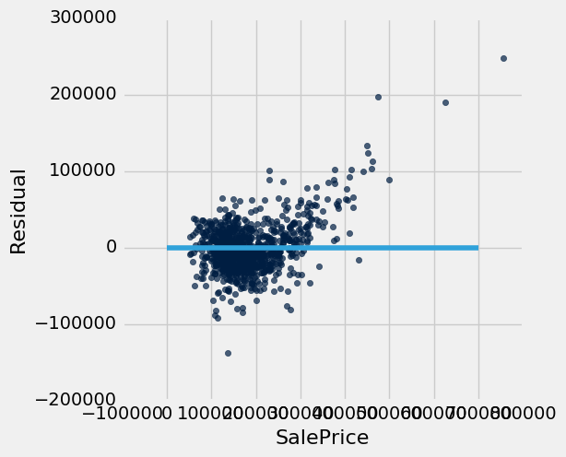## 最近邻回归

``````train_nn = train.select(0, 1, 2, 3, 4, 8)
test_nn = test.select(0, 1, 2, 3, 4, 8)
train_nn.show(3)
``````
SalePrice 1st Flr SF 2nd Flr SF Total Bsmt SF Garage Area Year Built
240000 1710 0 1710 550 2004
229000 1302 735 672 472 1996
136500 864 0 864 336 1978

（省略了 998 行）

``````def distance(pt1, pt2):
"""The distance between two points, represented as arrays."""
return np.sqrt(sum((pt1 - pt2) ** 2))

def row_distance(row1, row2):
"""The distance between two rows of a table."""
return distance(np.array(row1), np.array(row2))

def distances(training, example, output):
"""Compute the distance from example for each row in training."""
dists = []
attributes = training.drop(output)
for row in attributes.rows:
dists.append(row_distance(row, example))
return training.with_column('Distance', dists)

def closest(training, example, k, output):
"""Return a table of the k closest neighbors to example."""
return distances(training, example, output).sort('Distance').take(np.arange(k))

example_nn_row = test_nn.drop(0).row(0)
closest(train_nn, example_nn_row, 5, 'SalePrice')
``````
SalePrice 1st Flr SF 2nd Flr SF Total Bsmt SF Garage Area Year Built Distance
150000 1299 0 967 494 1954 51.9711
144000 1344 0 1024 484 1958 60.8358
183500 1299 0 1001 486 1979 68.6003
140000 1283 0 931 506 1962 76.5049
173000 1287 0 957 541 1977 77.2464

``````def predict_nn(example):
"""Return the majority class among the k nearest neighbors."""
return np.average(closest(train_nn, example, 5, 'SalePrice').column('SalePrice'))

predict_nn(example_nn_row)
158100.0
``````

``````print('Actual sale price:', test_nn.column('SalePrice').item(0))
print('Predicted sale price using nearest neighbors:', predict_nn(example_nn_row))
Actual sale price: 146000
Predicted sale price using nearest neighbors: 158100.0
``````

### 尾注

``````nn_test_predictions = test_nn.drop('SalePrice').apply(predict_nn)
rmse_nn = np.mean((test_prices - nn_test_predictions) ** 2) ** 0.5

print('Test set RMSE for multiple linear regression: ', rmse_linear)
print('Test set RMSE for nearest neighbor regression:', rmse_nn)
Test set RMSE for multiple linear regression:  30232.0744208
Test set RMSE for nearest neighbor regression: 31210.6572877
``````

``````test.with_column('Residual', test_prices-nn_test_predictions).scatter(0, 'Residual')
plots.plot([0, 7e5], [0, 0]);
``````